Download Presentation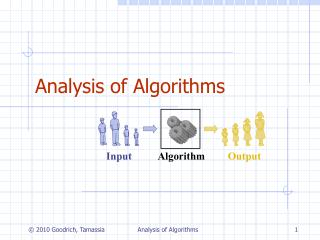Analysis of Algorithms

# Analysis of Algorithms - PowerPoint PPT Presentation

Analysis of Algorithms. Input. Algorithm. Output. Running Time. Most algorithms transform input objects into output objects. The running time of an algorithm typically grows with the input size. Average case time is often difficult to determine. We focus on the worst case running time.I am the owner, or an agent authorized to act on behalf of the owner, of the copyrighted work described.
Download Presentation## Analysis of Algorithms

An Image/Link below is provided (as is) to download presentation

Download Policy: Content on the Website is provided to you AS IS for your information and personal use and may not be sold / licensed / shared on other websites without getting consent from its author.While downloading, if for some reason you are not able to download a presentation, the publisher may have deleted the file from their server.

- - - - - - - - - - - - - - - - - - - - - - - - - - E N D - - - - - - - - - - - - - - - - - - - - - - - - - -
Presentation Transcript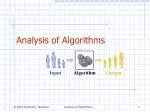### Analysis of Algorithms

Input

Algorithm

Output

Analysis of Algorithms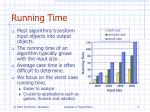Running Time
• Most algorithms transform input objects into output objects.
• The running time of an algorithm typically grows with the input size.
• Average case time is often difficult to determine.
• We focus on the worst case running time.
• Easier to analyze
• Crucial to applications such as games, finance and robotics

Analysis of Algorithms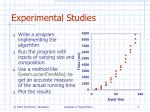Experimental Studies
• Write a program implementing the algorithm
• Run the program with inputs of varying size and composition
• Use a method like System.currentTimeMillis() to get an accurate measure of the actual running time
• Plot the results

Analysis of Algorithms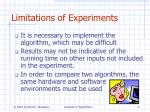Limitations of Experiments
• It is necessary to implement the algorithm, which may be difficult
• Results may not be indicative of the running time on other inputs not included in the experiment.
• In order to compare two algorithms, the same hardware and software environments must be used

Analysis of Algorithms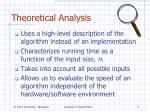Theoretical Analysis
• Uses a high-level description of the algorithm instead of an implementation
• Characterizes running time as a function of the input size, n.
• Takes into account all possible inputs
• Allows us to evaluate the speed of an algorithm independent of the hardware/software environment

Analysis of Algorithms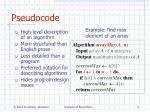Example: find max element of an array

AlgorithmarrayMax(A, n)

Inputarray A of n integers

Outputmaximum element of A

currentMaxA

fori1ton  1do

ifA[i]  currentMaxthen

currentMaxA[i]

returncurrentMax

Pseudocode
• High-level description of an algorithm
• More structured than English prose
• Less detailed than a program
• Preferred notation for describing algorithms
• Hides program design issues

Analysis of Algorithms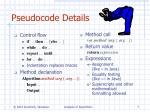Control flow

if…then… [else…]

while…do…

repeat…until…

for…do…

Indentation replaces braces

Method declaration

Algorithm method (arg [, arg…])

Input…

Output…

Method call

var.method (arg [, arg…])

Return value

returnexpression

Expressions

Assignment(like  in Java)

Equality testing(like  in Java)

n2 Superscripts and other mathematical formatting allowed

Pseudocode Details

Analysis of Algorithms2

1

0

The Random Access Machine (RAM) Model
• A CPU
• An potentially unbounded bank of memory cells, each of which can hold an arbitrary number or character

Memory cells are numbered and accessing any cell in memory takes unit time.

Analysis of Algorithms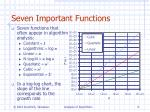Seven Important Functions
• Seven functions that often appear in algorithm analysis:
• Constant  1
• Logarithmic  log n
• Linear  n
• N-Log-N  n log n
• Quadratic  n2
• Cubic  n3
• Exponential  2n
• In a log-log chart, the slope of the line corresponds to the growth rate

Analysis of Algorithms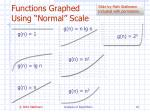Slide by Matt Stallmann included with permission.

Functions Graphed Using “Normal” Scale

g(n) = n lg n

g(n) = 1

g(n) = n2

g(n) = lg n

g(n) = n

g(n) = 2n

g(n) = n3

Analysis of AlgorithmsBasic computations performed by an algorithm

Identifiable in pseudocode

Largely independent from the programming language

Exact definition not important (we will see why later)

Assumed to take a constant amount of time in the RAM model

Examples:

Evaluating an expression

Assigning a value to a variable

Indexing into an array

Calling a method

Returning from a method

Primitive Operations

Analysis of AlgorithmsBy inspecting the pseudocode, we can determine the maximum number of primitive operations executed by an algorithm, as a function of the input size

AlgorithmarrayMax(A, n)

# operations

currentMaxA 2

fori1ton 1do 2n

ifA[i]  currentMaxthen 2(n 1)

currentMaxA[i] 2(n 1)

{ increment counter i } 2(n 1)

returncurrentMax 1

Total 8n 2

Counting Primitive Operations

Analysis of AlgorithmsAlgorithm arrayMax executes 8n 2 primitive operations in the worst case. Define:

a = Time taken by the fastest primitive operation

b = Time taken by the slowest primitive operation

Let T(n) be worst-case time of arrayMax.Thena (8n 2) T(n)b(8n 2)

Hence, the running time T(n) is bounded by two linear functions

Estimating Running Time

Analysis of AlgorithmsGrowth Rate of Running Time
• Changing the hardware/ software environment
• Affects T(n) by a constant factor, but
• Does not alter the growth rate of T(n)
• The linear growth rate of the running time T(n) is an intrinsic property of algorithm arrayMax

Analysis of AlgorithmsSlide by Matt Stallmann included with permission.

Why Growth Rate Matters

runtime

when problem

size doubles

Analysis of AlgorithmsSlide by Matt Stallmann included with permission.

Comparison of Two Algorithms

insertion sort is

n2 / 4

merge sort is

2 n lg n

sort a million items?

insertion sort takes

roughly 70 hours

while

merge sort takes

roughly 40 seconds

This is a slow machine, but if

100 x as fast then it’s 40 minutes

versus less than 0.5 seconds

Analysis of AlgorithmsConstant Factors
• The growth rate is not affected by
• constant factors or
• lower-order terms
• Examples
• 102n+105is a linear function
• 105n2+ 108nis a quadratic function

Analysis of AlgorithmsBig-Oh Notation
• Given functions f(n) and g(n), we say that f(n) is O(g(n))if there are positive constantsc and n0 such that

f(n)cg(n) for n n0

• Example: 2n+10 is O(n)
• 2n+10cn
• (c 2) n  10
• n  10/(c 2)
• Pick c = 3 and n0 = 10

Analysis of AlgorithmsBig-Oh Example
• Example: the function n2is not O(n)
• n2cn
• n c
• The above inequality cannot be satisfied since c must be a constant

Analysis of AlgorithmsMore Big-Oh Examples

7n-2

7n-2 is O(n)

need c > 0 and n0 1 such that 7n-2  c•n for n  n0

this is true for c = 7 and n0 = 1

• 3n3 + 20n2 + 5

3n3 + 20n2 + 5 is O(n3)

need c > 0 and n0 1 such that 3n3 + 20n2 + 5  c•n3 for n  n0

this is true for c = 4 and n0 = 21

• 3 log n + 5

3 log n + 5 is O(log n)

need c > 0 and n0 1 such that 3 log n + 5  c•log n for n  n0

this is true for c = 8 and n0 = 2

Analysis of AlgorithmsBig-Oh and Growth Rate
• The big-Oh notation gives an upper bound on the growth rate of a function
• The statement “f(n) is O(g(n))” means that the growth rate of f(n) is no more than the growth rate of g(n)
• We can use the big-Oh notation to rank functions according to their growth rate

Analysis of AlgorithmsBig-Oh Rules
• If is f(n) a polynomial of degree d, then f(n) is O(nd), i.e.,
• Drop lower-order terms
• Drop constant factors
• Use the smallest possible class of functions
• Say “2n is O(n)”instead of “2n is O(n2)”
• Use the simplest expression of the class
• Say “3n+5 is O(n)”instead of “3n+5 is O(3n)”

Analysis of AlgorithmsAsymptotic Algorithm Analysis
• The asymptotic analysis of an algorithm determines the running time in big-Oh notation
• To perform the asymptotic analysis
• We find the worst-case number of primitive operations executed as a function of the input size
• We express this function with big-Oh notation
• Example:
• We determine that algorithm arrayMax executes at most 8n 2 primitive operations
• We say that algorithm arrayMax “runs in O(n) time”
• Since constant factors and lower-order terms are eventually dropped anyhow, we can disregard them when counting primitive operations

Analysis of AlgorithmsComputing Prefix Averages
• We further illustrate asymptotic analysis with two algorithms for prefix averages
• The i-th prefix average of an array X is average of the first (i+ 1) elements of X:

A[i]= (X +X +… +X[i])/(i+1)

• Computing the array A of prefix averages of another array X has applications to financial analysis

Analysis of AlgorithmsThe following algorithm computes prefix averages in quadratic time by applying the definition

AlgorithmprefixAverages1(X, n)

Inputarray X of n integers

Outputarray A of prefix averages of X #operations

A new array of n integers n

fori0ton 1do n

sX n

forj1toido 1 + 2 + …+ (n 1)

ss+X[j] 1 + 2 + …+ (n 1)

A[i]s/(i+ 1)n

returnA 1

Analysis of AlgorithmsThe running time of prefixAverages1 isO(1 + 2 + …+ n)

The sum of the first n integers is n(n+ 1) / 2

There is a simple visual proof of this fact

Thus, algorithm prefixAverages1 runs in O(n2) time

Arithmetic Progression

Analysis of AlgorithmsPrefix Averages (Linear)

The following algorithm computes prefix averages in linear time by keeping a running sum

AlgorithmprefixAverages2(X, n)

Inputarray X of n integers

Outputarray A of prefix averages of X #operations

A new array of n integers n

s 0 1

fori0ton 1do n

ss+X[i] n

A[i]s/(i+ 1)n

returnA 1

Algorithm prefixAverages2 runs in O(n) time

Analysis of AlgorithmsMath you need to Review

Summations

Logarithms and Exponents

Proof techniques

Basic probability

• properties of logarithms:

logb(xy) = logbx + logby

logb (x/y) = logbx - logby

logbxa = alogbx

logba = logxa/logxb

• properties of exponentials:

a(b+c) = aba c

abc = (ab)c

ab /ac = a(b-c)

b = a logab

bc = a c*logab

Analysis of AlgorithmsRelatives of Big-Oh

• big-Omega
• f(n) is (g(n)) if there is a constant c > 0

and an integer constant n0  1 such that

f(n)  c•g(n) for n  n0

• big-Theta
• f(n) is (g(n)) if there are constants c’ > 0 and c’’ > 0 and an integer constant n0  1 such that c’•g(n)  f(n)  c’’•g(n) for n  n0

Analysis of AlgorithmsIntuition for Asymptotic Notation

Big-Oh

• f(n) is O(g(n)) if f(n) is asymptotically less than or equal to g(n)

big-Omega

• f(n) is (g(n)) if f(n) is asymptotically greater than or equal to g(n)

big-Theta

• f(n) is (g(n)) if f(n) is asymptotically equal to g(n)

Analysis of AlgorithmsExample Uses of the Relatives of Big-Oh

• 5n2 is (n2)

f(n) is (g(n)) if there is a constant c > 0 and an integer constant n0  1 such that f(n)  c•g(n) for n  n0

let c = 5 and n0 = 1

• 5n2 is (n)

f(n) is (g(n)) if there is a constant c > 0 and an integer constant n0  1 such that f(n)  c•g(n) for n  n0

let c = 1 and n0 = 1

• 5n2 is (n2)

f(n) is (g(n)) if it is (n2) and O(n2). We have already seen the former, for the latter recall that f(n) is O(g(n)) if there is a constant c > 0 and an integer constant n0  1 such that f(n) <c•g(n) for n  n0

Let c = 5 and n0 = 1

Analysis of Algorithms